Density of states

﻿
Density of states

In solid-state and condensed matter physics, the density of states (DOS) of a system describes the number of states per interval of energy at each energy level that are available to be occupied by electrons. Unlike isolated systems, like atoms or molecules in gas phase, the density distributions are not discrete like a spectral density but continuous. A high DOS at a specific energy level means that there are many states available for occupation. A DOS of zero means that no states can be occupied at that energy level. In general a DOS is an average over the space and time domains occupied by the system. Local variations, most often due to distortions of the original system, are often called local density of states (LDOS). If the DOS of an undisturbed system is zero, the LDOS can locally be non-zero due to the presence of a local potential.

Introduction

In quantum mechanical (QM) systems, not all waves, or wave-like particles, are allowed to exist. In some systems, the interatomic spacing and the atomic charge of the material allows only electrons of certain wavelengths to exist. In other systems, the crystalline structure of the material allows waves to propagate in one direction, while suppressing wave propagation in another direction. The most familiar systems, like neutronium in neutron stars and free electron gases in metals (examples of degenerate matter and a Fermi gas), have a 3-dimensional Euclidean topology. Less familiar systems, like 2-dimensional electron gases (2DEG) in graphite layers and the Quantum Hall effect system in MOSFET type devices, have a 2-dimensional Euclidean topology. Even less familiar are Carbon nanotubes, the quantum wire and Luttinger liquid with their 1-dimensional topologies. The topological properties of the system have a major impact on the properties of the density of states. Systems with 1D and 2D topologies are likely to become more common, assuming developments in nanotechnology and materials science proceed. Waves in a QM system have specific wavelengths and can propagate in specific directions, and each wave occupies a different mode, or state. Because many of these states have the same wavelength, and therefore share the same energy, there may be many states available at certain energy levels, while no states are available at other energy levels.

For example, the density of states for electrons in a semiconductor is shown in red in Fig. 4. For electrons at the conduction band edge, very few states are available for the electron to occupy. As the electron increases in energy, the electron density of states increases and more states become available for occupation. However, because there are no states available for electrons to occupy within the bandgap, electrons at the conduction band edge must lose at least Eg of energy in order to transition to another available mode. The density of states can be calculated for electron, photon, or phonon in QM systems. The DOS is usually represented by one of the symbols g, ρ, D, n, or N, and can be given as a function of either energy or wave vector k. To convert between energy and wave vector, the specific relation between E and k must be known.

Symmetry and density of states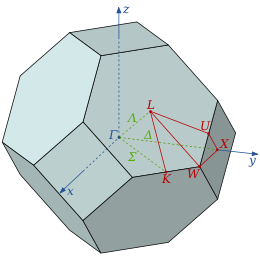First Brillouin zone of FCC lattice, a truncated octahedron, showing symmetry labels for high symmetry lines and points

There are a large variety of systems and types of states for which DOS calculations can be done. An important property of a condensed matter system is the symmetry of the structure on its microscopic scale. Fluids, glasses or amorphous solids have dispersion relations with a rotational symmetry. In spherically symmetric systems the integrals of functions, for instance, are one-dimensional because all variables in the calculation depend only on the radial parameter of the dispersion relation.

Angular dependent calculations or measurements on a systems consisting of a single crystal of a compound, for example, are not anisotropic. The DOS in such cases is often called a projected density of states. Evaluation and calculation of functions and integrals are done on fixed points and in a certain direction and along certain lines or planes through the anisotropic dispersion relations of the system.Octahedron.

Measurements on powders or polycrystalline samples require evaluation and calculation functions and integrals over the whole domain, most often a Brillouin zone, of the dispersion relations the system of interest. Sometimes the symmetry of the system is high. The shape of the functions describing the dispersion relations of the system appears many times over the whole domain of the dispersion relation. In such cases the effort to calculate the DOS can be reduced by a great amount when the calculation is limited to a reduced zone or fundamental domain. The Brillouin zone of the FCC lattice in the figure on the right has the 48 fold symmetry of the point group Oh with full octahedral symmetry. This means that the integration over the whole domain of the Brillouin zone can be reduced to a 48-th part of the whole Brillouin zone. As a crystal structure periodic table shows, there are many elements with a FCC crystal structure, like Diamond, Silicon and Platinum and their Brillouin zones and dispersion relations have this 48 fold symmetry.First Brillouin zone of BCC lattice, a rhombic dodecahedron.

Two other familiar crystal structures are the BCC and HCP structures with cubic and hexagonal lattices. The BCC structure has the 24 fold pyritohedral symmetry of the point group Th. The HCP structure has the 12 fold prismatic dihedral symmetry of the point group D3h. A complete list of symmetry properties of a point group can be found in point group character tables.

In general it is easier to calculate a DOS when the symmetry of the system is higher and the number of topological dimensions of the dispersion relation is lower. The DOS of dispersion relations with rotational symmetry can often be calculated analytically. In this respect nature is at the hand of calculating and analyzing man because systems with high symmetry are thermodynamically favorable over systems with lower symmetry.

This is because the entropy of a system of higher symmetry, like the case of a higher so called multiplicity, is also higher. The thermally excited particles in the system, with some arbitrary energy in a partially filled band, can be distributed over more available states. There are more possible ways to distribute them because there are more states available what results in a higher entropy.

k-space topologies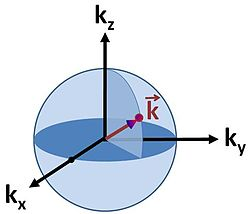Figure 1: Spherical surface in k-space for electrons in three dimensions.

The density of states is dependent upon the dimensional limits of the object itself. The role dimensions play is evident from the units of DOS (Energy−1Volume−1). In the limit that the system is 2 dimensional a volume becomes an area and in the limit of 1 dimension it becomes a length. It is important to note that the volume being referenced is the volume of k-space, the space enclosed by the constant energy surface of the system derived through a dispersion relation that relates E to k. The dispersion relation for electrons in a solid is given by the electronic band structure. An example of a 3-dimensional k-space is given in Fig. 1. It can be seen that the dimensionality of the system itself will confine the momentum of particles inside the system.

Density of wave vector states

The calculation for DOS starts by counting the N allowed states at a certain k that are contained within [k, k+dk] inside the volume of the system. This is done by differentiating the whole k-space volume Ωn,k in n-dimensions at an arbitrary k, with respect to k. The volume, area or length in 3, 2 or 1-dimensional k-spaces are expressed by

Ωn(k) = cnkn

for a n-dimensional k-space with the topologically determined constants$c_1 = 2,\text{ } c_2 = \pi,\text{ } c_3 = \frac{4 \pi}{3}$

for linear, disk and spherical symmetrical shaped functions in 1, 2 and 3-dimensional Euclidian k-spaces respectively.

According to this scheme the density of wave vector states N is, by differentiating Ωn,k with respect to k, expressed by$N_n(k) = \frac{d\Omega_n(k)}{dk} = n\text{ } c_n \text{ } k^{(n - 1)}$

The 1, 2 and 3-dimensional density of wave vector states are explicitly written as

N1(k) = 2
N2(k) = 2πk
N3(k) = 4πk2

One state is large enough to contain particles having wavelength λ. The wavelength is related to k through the relationship.$k = \frac{2\pi}{\lambda}$

In a quantum system the length of λ will depend on a characteristic spacing of the system L that is confining the particles. Finally the density of states N is multiplied by a factor s, where s is a constant degeneracy factor that accounts for internal degrees of freedom due to such physical phenomena as spin or polarization. If no such phenomenon is present then s = 1. Vk is the volume in k-space containing all states whose wavevectors are smaller than a certain k serving as a parameter.

Density of energy states

To finish the calculation for DOS find the number of states per unit sample volume at an energy E inside an interval [E,E + dE]. The general form of DOS of a system is given as$D_n\left(E\right) = \frac{d\Omega_n(E)}{dE}$

The scheme sketched so far only applies to continuously rising and spherically symmetric dispersion relations. In general the dispersion relation E(k) is not spherically symmetric and in many cases it isn't continuously rising either. To express D as a function of E the inverse of the dispersion relation E(k) has to be substituted into the expression of Ωn(k) as a function of k to get the expression of Ωn(E) as a function of the energy. If the dispersion relation is not spherically symmetric or continuously rising and can't be inverted easily then in most cases the DOS has to be calculated numerically. More detailed derivations are available.

Dispersion relations

The kinetic energy of a particle depends on the magnitude and direction of the wave vector k, the properties of the particle and the environment in which the particle is moving. For example, the kinetic energy of an electron in a Fermi gas is given by$E = E_0 + \frac{(\hbar k)^2}{2m} \ ,$

where m is the electron mass. The dispersion relation is a spherically symmetric parabola and it is continuously rising so the DOS can be calculated easily.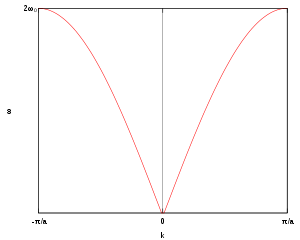Figure 2: Monoatomic chain phonon dispersion relation

For longitudinal phonons in a string of atoms the dispersion relation of the kinetic energy in a 1-dimensional k-space, as shown in Figure 2, is given by$E = 2 \hbar \omega_0 |\sin(ka/2)|$

where$\omega_0 = \sqrt{k_F / m}$ is the oscillator frequency, m the mass of the atoms, kF the inter-atomic force constant and a inter-atomic spacing. For small values of k < < π / a the dispersion relation is rather linear:$E = \hbar \omega_0 ka$

When$k \approx \pi / a$ the energy is$E = 2 \hbar \omega_0 |\cos(\pi/2 - ka/2)|$

With the transformation q = k − π / a and small q this relation can be transformed to$E = 2 \hbar \omega_0 [1 - (qa/2)^2]$

Isotropic dispersion relations

The two examples mentioned here can be expressed like

E = E0 + ckkp

This is a kind of dispersion relation because it interrelates two wave properties and it is isotropic because only the length and not the direction of the wave vector appears in the expression. The magnitude of the wave vector is related to the energy as:$k = \left(\frac{E-E_0}{c_k}\right)^{1/p} \ ,$

Accordingly, the volume of n-dimensional k-space containing wave vectors smaller than k is:

Ωn(k) = cnkn

Substitution of the isotropic energy relation gives the volume of occupied states$\Omega_n(E) = \frac{c_n}{c_k^{n/p}}\left(E-E_0\right)^{n/p}\ ,$

Differentiating this volume with respect to the energy gives an expression for the DOS of the isotopic dispersion relation$D_n\left(E\right) = \frac {d}{dE}\Omega_n(E) = \frac{n c_n}{p c_k^{n/p}}\left(E-E_0\right)^{(n/p - 1)}$

Parabolic dispersion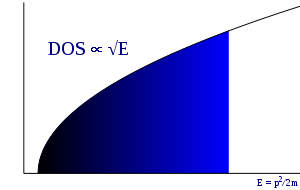Figure 3: Free-electron DOS in 3-dimensional k-space

In the case of a parabolic dispersion relation (p = 2), such as applies to free electrons in a Fermi gas, the resulting density of states,$D_n\left(E\right)$, for electrons in a n-dimensional systems is$D_1\left(E\right) = \frac {1}{ \sqrt{c_k(E-E_0)}} \ .$$D_2\left(E\right) = \frac {\pi}{c_k}$$D_3\left(E\right) = 2 \pi \sqrt{\frac{E-E_0}{c_k^3}} \ .$

In 1-dimensional systems the DOS diverges at the bottom of the band as E drops to E0. In 2-dimensional systems the DOS turns out to be independent of E. Finally for 3-dimensional systems the DOS rises as the square root of the energy.

Linear dispersion

In the case of a linear relation (p = 1), such as applies to photons, or to some special kinds of electronic bands in a solid, the DOS in 1, 2 and 3 dimensional systems is related to the energy as:$D_1\left(E\right) = \frac{1}{c_k}$$D_2\left(E\right) = \frac{2 \pi}{c_k^2}\left(E-E_0\right)$$D_3\left(E\right) = \frac{4 \pi}{c_k^3}\left(E-E_0\right)^2$

Density of states and distribution functions

The density of states plays an important role in the kinetic theory of solids. The product of the density of states and the probability distribution function is the number of occupied states per unit volume at a given energy for a system in thermal equilibrium. This value is widely used to investigate various physical properties of matter. The following are examples, using two common distribution functions, of how applying a distribution function to the density of states can give rise to physical properties.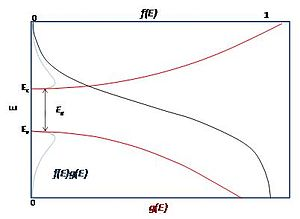Figure 4: The density of states (red), the Fermi-Dirac probability distribution (black), and their product (blue) for a semiconductor. The valence band and the conduction band are labeled on the ordinate.

Fermi-Dirac statistics: The Fermi-Dirac probability distribution function, Fig. 4, is used to find the probability that a fermion occupies a specific quantum state in a system at thermal equilibrium. Fermions are particles which obey the Pauli Exclusion Principle (e.g. electrons, protons, neutrons). The distribution function can be written as$f_{\mathrm{FD}}\left(E\right) = \frac{1}{\exp\left(\frac{E-\mu}{k_BT}\right)+1}$

μ is the chemical potential (also denoted as EF and called the Fermi level), kB is the Boltzmann constant, and T is temperature. Fig. 4 illustrates how the product of the Fermi-Dirac distribution function and the three dimensional density of states for a semiconductor can give insight to physical properties such as carrier concentration and Energy band gaps.

Bose-Einstein statistics: The Bose-Einstein probability distribution function is used to find the probability that a boson occupies a specific quantum state in a system at thermal equilibrium. Bosons are particles which do not obey the Pauli Exclusion Principle (e.g. phonons and photons). The distribution function can be written as$f_{\mathrm{BE}}\left(E\right) = \frac{1}{\exp\left(\frac{E-\mu}{k_BT}\right)-1}$

From these two distributions it is possible to calculate properties such as the internal energy U, the density of particles n, specific heat capacity C, and thermal conductivity k. The relationships between these properties and the product of the density of states and the probability distribution, denoting the density of states by$g\left(E\right)$ instead of$D\left(E\right)$, are given by$U = \int E f\left(E\right)g\left(E\right)\,dE$$n = \int f(E)g(E)\,dE$$C = \frac{\partial}{\partial T}\int E f\left(E\right)g\left(E\right)\,dE$$k = \frac{1}{d}\frac{\partial}{\partial T}\int E f\left(E\right)g\left(E\right)\nu\left(E\right)\Lambda\left(E\right)\,dE$

d is dimensionality, ν is sound velocity and Λ is mean free path.

Applications

The density of states appears in many areas of physics, and helps to explain a number of quantum mechanical phenomena.

Quantization

Calculating the density of states for small structures shows that the distribution of electrons changes as dimensionality is reduced. For quantum wires, the DOS for certain energies actually becomes higher than the DOS for bulk semiconductors, and for quantum dots the electrons become quantized to certain energies.

Photonic crystals

The photon density of states can be manipulated by using periodic structures with length scales on the order of the wavelength of light. Some structures can completely inhibit the propagation of light of certain colors (energies), creating a photonic bandgap: the DOS is zero for those photon energies. Other structures can inhibit the propagation of light only in certain directions to create mirrors, waveguides, and cavities. Such periodic structures are known as photonic crystals.

Calculation of the density of states

Interesting systems are in general complex, for instance compounds, biomolecules, polymers, etc. Because of the complexity of these systems the analytical calculation of the density of states is in most of the cases impossible. Computer simulations offer a set of algorithms to evaluate the density of states with a high accuracy. One of these algorithms is called the Wang and Landau algorithm.

Within the Wang and Landau scheme any previous knowledge of the density of states is required. One proceed as follows: the cost function (for example the energy) of the system is discretized. Each time the bin i is reached one update a histogram for the density of, states g(i),by,$g(i) \rightarrow g(i) + f ,$

where f is called modification factor. As soon as each bin in the histogram is visited a certain number of times (10-15), the modification factor is reduced by some criterion, for instance,$f_{n+1} \rightarrow (1/2)f_{n}$

where n denotes the n-th update step. The simulation finishes when the modification factor is less than a certain threshold, for instance fn < 10 − 8.

The Wang and Landau algorithm has some advantages over other common algorithms such as multicanonical simulations and Parallel tempering, for instance, the density of states is obtained as the main product of the simulation. Additionally, Wang and Landau simulations are completely independent of the temperature. This feature allows to compute the density of states of systems with very rough energy landscape such as proteins.

 Effective mass (solid-state physics) Band structure k·p perturbation theory Semiconductor Electrical conduction Valence band Kronig-Penney model Tight-binding model Muffin-tin approximation

Wikimedia Foundation. 2010.

Look at other dictionaries:

• density of states — būsenų tankis statusas T sritis Standartizacija ir metrologija apibrėžtis Apibrėžtį žr. priede. priedas( ai) Grafinis formatas atitikmenys: angl. density of states; state density vok. Zustandsdichte, f rus. плотность состояний, f; плотность числа …   Penkiakalbis aiškinamasis metrologijos terminų žodynas

• Local density of states — (LDOS) is a physical quantity that describes the density of states, but space resolved. In materials science, this term is useful when interpreting the data from STM, since this method is capable of imaging electron densities of states with… …   Wikipedia

• Density (disambiguation) — Density and dense usually refer to a measure of how much of some entity is within a fixed amount of space. Types of density include: In physics, density of mass: Density, mass per volume Area density or surface density, mass over a (two… …   Wikipedia

• Density altitude — Computation Chart. Density altitude is the altitude in the International Standard Atmosphere at which the air density would be equal to the actual air density at the place of observation, or, in other words, the height when measured in terms of… …   Wikipedia

• Density matrix — Mixed state redirects here. For the psychiatric condition, see Mixed state (psychiatry). In quantum mechanics, a density matrix is a self adjoint (or Hermitian) positive semidefinite matrix (possibly infinite dimensional) of trace one, that… …   Wikipedia

• Density functional theory — Electronic structure methods Tight binding Nearly free electron model Hartree–Fock method Modern valence bond Generalized valence bond Møller–Plesset perturbation theory …   Wikipedia

• Density — This article is about mass density. For other uses, see Density (disambiguation). The mass density or density of a material is defined as its mass per unit volume. The symbol most often used for density is ρ (the Greek letter rho). In some cases… …   Wikipedia

• Density matrix renormalization group — The density matrix renormalization group (DMRG) is a numerical variational technique devised to obtain the low energy physics of quantum many body systems with high accuracy. It was invented in 1992 by Steven R. White and it is nowadays the most… …   Wikipedia

• Density theorem for Kleinian groups — In the mathematical theory of Kleinian groups, the density conjecture of Lipman Bers, Dennis Sullivan, and William Thurston, states that every finitely generated Kleinian group is an algebraic limit of geometrically finite Kleinian groups.… …   Wikipedia

• States of Austria — Austria is a federal republic made up of nine states, known in German as Länder (singular Land ). Since Land is also the German word for country , the term Bundesländer ( Federal States ; singular Bundesland ) is often used instead to avoid… …   Wikipedia